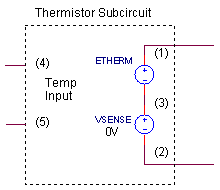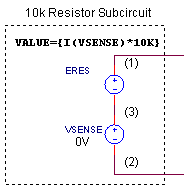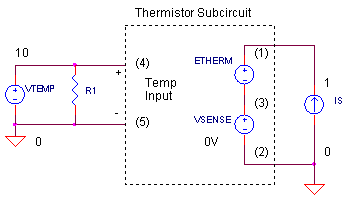eCircuit  Center

### NTC Thermistor Model

CIRCUITOf the many choices for temperature sensing - thermocouple, RTD, thermistor - the thermistor serves up one big advantage: a large signal. This means you don't have to amplify it as much as the other sensors (and amplify all that goes with the signal such as noise, offsets, drifts, etc.) But, as we've come to expect, for every advantage there's at least one disadvantage; the thermistor is highly non-linear. This poses the questions of not only how do we process the signal, but, how do we model it in SPICE?

We'll have to move beyond the basic RLC components to emulate the thermistor's non-linear behavior. But, once the equation describing a thermistor's resistance versus temperature is known, creating a model is easier than you think. To get there, we just need a simple trick of modeling a resistor without using a resistor. Then, an equation that describes the resistance as a function of temperature will be put in the model.

THERMISTOR RESISTANCE

How does a thermistor behave versus temperature? As temperature increases, its resistance gets smaller according to the R vs. T equation

R = Ro exp( Beta/T - Beta/To)

where

 R Thermistor resistance at T (K) T Thermistor temperature (K) Ro Nominal resistance at To (K) To Temperature where Ro is measured Beta Thermistor material constant

This exponential equation describes a very non-linear curve that decreases with increasing temperature. For this reason, you may see the thermistor referred to as a Negative Temperature Coefficient (NTC) device. As an example, suppose you purchased a thermistor that measures Ro = 10k at To = 25 and has a material constant Beta = 3548. The device should measure resistances across a -15 to 65 deg C range that look like

 T (deg C) T (K) = T(deg C) + 273 R (Ohms) -15 258 63338 +25 298 10000 +65 338 2444

A RESISTOR MODEL WITHOUT A RESISTOR

An important step in modeling a thermistor is creating a model of a resistor without using a resistor. But how? Think of the resistor's behavior when current is passed through it. A voltage is produced according to V = I x R. Place the resistor in the circuit - it does the job automatically. But, let's rip out the resistor and do the job manually using other SPICE components. In its place, simply sense the current I and generate the voltage V = I x R with a voltage source. Here's a subcircuit that models a 10k resistor..SUBCKT RES_10K 1 2
ERES 1 3 VALUE = { I(VSENSE)*10K }
VSENSE 3 2 DC 0
.ENDS

To sense current, SPICE uses a voltage source VSENSE (0V). It's set to 0V so there's no effect on the output voltage. The other source, ERES, generates the voltage across the "resistor" based on the sensed current times your desired resistance: VALUE={ I(VSENSE)* 10K }. The beauty of this source is that its output voltage can be described by an equation! Wow, think of the possibilities! Too bad real world components weren't just as easy to create. But at least we have that power when simulating them in SPICE.

THERMISTOR SPICE MODEL

To create the thermistor model, just extend the resistor's subcircuit above to include the R vs. T equation and the sensor's temperature.

.SUBCKT NTC_10K_1   1 2   4 5
ETHERM 1 3 VALUE = { I(VSENSE)*10K*EXP( 3548/(V(4,5)+273) - 3548/(25+273) ) }
VSENSE 3 2 DC 0
.ENDS

As you can see, nodes 4 and 5 have been added. This is the sensor's temperature in Volts (but we know it represents degrees Celsius). And finally, the R vs. T equation has been included in the ETHERM statement to create our compact, but powerful, sensor model. Notice the temperature,  V(4,5), included in the equation.

THERMISTOR TEST

How do you measure the resistance of a component using SPICE? Injecting a 1 A current source through a device and measuring the voltage across it produces a plot that really represents the resistance (V = I x R = 1 x R = R). But remember, although this technique is handy for SPICE, it's impractical for real components on the bench. (A 1A current may toast the component or attempt to generate large voltages beyond the limits of the current source or oscilloscope. Scaling down the current to the mA range is more reasonable on the bench.)CIRCUIT ANALYSIS   Run a simulation of the SPICE file THERM_MODEL1.CIR. Current source IS generates the 1A source for the resistance measurement. Plot the thermistor's resistance V(1) (really in ohms). Does your simulation match the expected values at -15, +25 and +65 deg C as listed in the table above?

Notice how voltage source VTEMP generates the sensor's temperature. It contains a Piece-Wise-Linear (PWL) statement that creates a voltage ramping linearly from -15V at 0 ms to 65 V at 100ms. Although it's a voltage, we know it represents temperature. We'll even throw in the DEG units in the PWL statement, just as a reminder, which SPICE ignores anyway.

HANDS-ON DESIGN   Manufacturer's typically make thermistors available at various Ro values with the same material constant. Suppose the 10k thermistor also comes in another resistance like 1k. To simulate it, change the 10k in the VALUE statement to 1k. Rerun the simulation. The R vs. T curve should be scaled by a factor of 10 lower than before. Did the curve keep its shape as before? It should. However, the shape will change given a different material constant. To see its effect on the shape, pick a different Beta (initially 3548) and plot the new curve.

FINAL NOTE

The next part of this story is how to linearize this exponential curve in your temperature range of interest. See the Linearized Thermistor circuit.

Check out several other Voltage-Controlled Resistor models.

Review SPICE Subcircuits at the SPICE Basics page.

SPICE FILE

Download the file or copy this netlist into a text file with the *.cir extention.

```THERM_MODEL1.CIR - THERMISTOR NTC MODEL
*
* SENSOR TEMPERATURE
VTEMP	10	0	PWL(0MS -15DEG   100MS 65DEG)
R1		10	0	1MEG
*
* CURRENT SOURCE TO MEASURE R
IS	0	1	DC	1
*
* DEVICE UNDER TEST
XTH1	1 0	10 0   NTC_10K_1
*XTH1	1       0	RES_10K
*
*
* THERMISTOR SUBCIRCUIT
*
* thermistor terminals:	1,2
* temperature input+,-:	4,5
* (temperature in deg C)
*
.SUBCKT NTC_10K_1   1  2  4  5
ETHERM	1 3	 VALUE = { I(VSENSE)*10K*EXP( 3548/(V(4,5)+273) - 3548/(25+273) ) }
VSENSE	3	2	DC	0
.ENDS
*
*
* RESISTOR SUBCIRCUIT
*
* resistor's terminals:	1,2
*
.SUBCKT RES_10K   1  2
ERES	1 3	 VALUE = { I(VSENSE)*10K }
VSENSE	3	2	DC	0
.ENDS
*
* ANALYSIS
.TRAN 	0.1MS  100MS
* VIEW RESULTS
.PRINT	TRAN 	V(1)
.PROBE
.END```

top